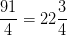# SSAT Upper Level Math : How to find out a mixed fraction from an improper fraction

## Example Questions

### Example Question #1 : How To Find Out A Mixed Fraction From An Improper Fraction

Which of the following is one more than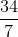?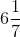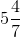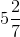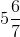Explanation:

Rewriteas follows: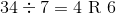, so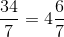.

Add one: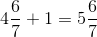### Example Question #2 : How To Find Out A Mixed Fraction From An Improper Fraction

Which of the following is three times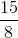?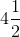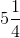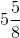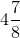Explanation: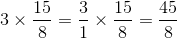Since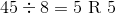,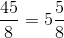### Example Question #3 : How To Find Out A Mixed Fraction From An Improper Fraction

Which of the following is 80% of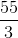?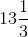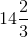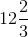Explanation:

80% of a number is equal tomultiplied by that number, so 80% ofis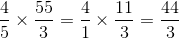Since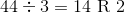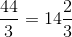.

### Example Question #4 : How To Find Out A Mixed Fraction From An Improper Fraction

Which of the following is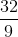increased by 25%?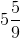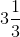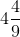The correct answer is not among the other responses.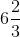Explanation:

A number increased by 25% is 125%, or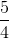, multiplied by the number.increased by 25% is therefore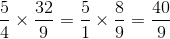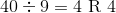, so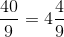### Example Question #5 : How To Find Out A Mixed Fraction From An Improper Fraction

Decrease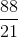by 12.5%. What is the result?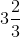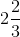Explanation:

Decreasing a number by 12.5% is the same as taking 87.5% of a number, or multiplying it by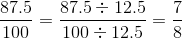.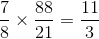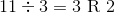, so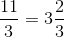### Example Question #6 : How To Find Out A Mixed Fraction From An Improper Fraction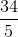Change to a mixed number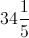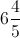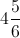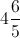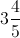Explanation:

To convert from a fraction to a mixed number we must find out how many times the denominator goes into the numerator using division and the remainder becomes the new fraction.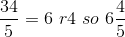### Example Question #7 : How To Find Out A Mixed Fraction From An Improper Fraction

Write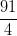as a mixed fraction.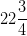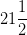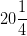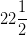Explanation:

Divide the numerator by the denominator.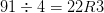Now, the whole number part will be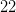. Write the remainder on top of the denominator to get the fraction.